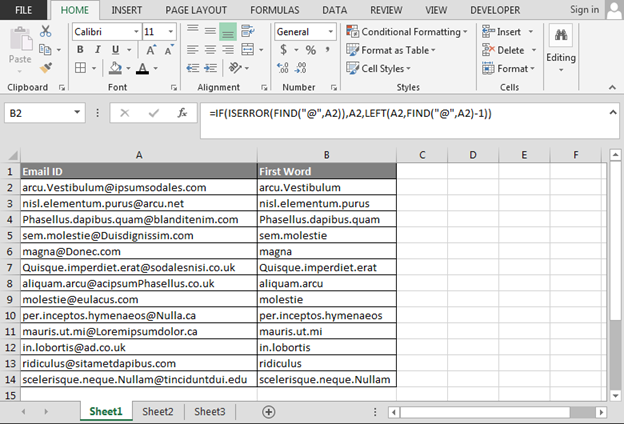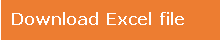# Excel formula to extract the first word in a cell

In this article, we are going to learn Excel formula which we can use to get the first word from a cell.

To resolve this query, we will use IF, ISERROR, FIND and LEFT functions.

In this situation, IF function will help to check the multiple conditions at one time and will help to get the result.

ISERROR function will help to give the situation if result is any error.

FIND function will help to find the symbol after first word.

LEFT function will pick the value from the left side.

Let’s take an example to understand.

We have email id’s data in Excel, and we want to retrieve the first word or name from the email id’s.Follow below steps and formula:-

• Enter the formula in cell B2.
• =IF(ISERROR(FIND("@",A2)),A2,LEFT(A2,FIND("@",A2)-1))
• Press Enter.
• Copy formula in the range.• First Name or first word will be extracted from the column.

Note:- If you want to extract first word before the space, then you just replace @ with space in the formula, and then you can apply.

This is the way we can make the customize formula according to our data and requirement in Microsoft Excel.## Comments

1."I am trying to write a function to remove the first part of a string of words (i.e ""who,what,when,where"") but with no luck.
I can use the formula :-
=IF(ISERR(FIND("","",A1)),A1,LEFT(A1,FIND("","",A1)-1)) , in a cell but need to write a function in the VB editor to do the same thing!
Does anyone have any ideas?
Regards"

Terms and Conditions of use

The applications/code on this site are distributed as is and without warranties or liability. In no event shall the owner of the copyrights, or the authors of the applications/code be liable for any loss of profit, any problems or any damage resulting from the use or evaluation of the applications/code.